Function Repository Resource:

# EveryOther

Split a list into two sublists, gathering every other element of the list

Contributed by: Wolfram Staff
 ResourceFunction["EveryOther"][{e1,e2,e3,e4,…}] returns {{e1,e3,…},{e2,e4,…}}. ResourceFunction["EveryOther"][{e1,e2,…},n] returns n sublists {{e1,e1+n,…},…{en,e2n,…}}.

## Details and Options

For a list of even length, ResourceFunction["EveryOther"][list] is equivalent to Transpose[Partition[list,2]].
If n evenly divides the list length, ResourceFunction["EveryOther"][list,n] is equivalent to Transpose[Partition[list,n]].

## Examples

### Basic Examples (2)

Split the List, combining elements in odd and even positions:

 In:=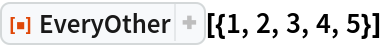Out=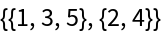Group elements separated by gaps of length n:

 In:=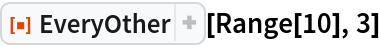Out=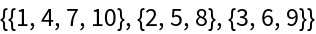### Properties and Relations (2)

Transpose[Partition[list,2]] gives the same result as EveryOther[list], as long as list has an even number of elements:

 In:=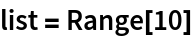Out=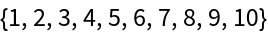In:=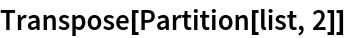Out=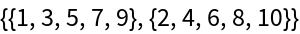In:=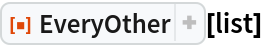Out=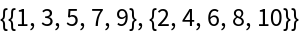Transpose[Partition[list,n]] gives the same result as EveryOther[list,n], as long n evenly divides Length[list]:

 In:=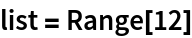Out=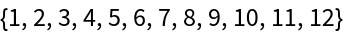In:=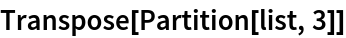Out=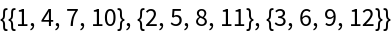In:=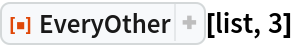Out=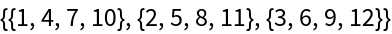## Version History

• 1.0.0 – 31 August 2020